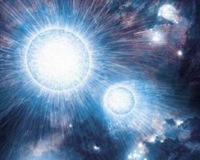Cococubed.com Colliding White Dwarfs

Home

Astronomy research
Software Infrastructure:
MESA
FLASH
STARLIB
MESA-Web
starkiller-astro
My instruments
White dwarf supernova:
Remnant metallicities
Colliding white dwarfs
Merging white dwarfs
Ignition conditions
Metallicity effects
Central density effects
Detonation density effects
Tracer particle burning
Subsonic burning fronts
Supersonic burning fronts
W7 profiles
Massive star supernova:
Rotating progenitors
3D evolution
26Al & 60Fe
44Ti, 60Co & 56Ni
Effects of 12C +12C
SN 1987A light curve
Constraints on Ni/Fe ratios
An r-process
Neutron Stars and Black Holes:
Black Hole Mass Gap
Compact object IMF
Stars:
Neutrino HR diagram
Pulsating white dwarfs
Pop III with JWST
Monte Carlo massive stars
Neutrinos from pre-SN
Pre-SN variations
Monte Carlo white dwarfs
SAGB stars
Classical novae
He shell convection
Presolar grains
He burn on neutron stars
BBFH at 40 years
Chemical Evolution:
Iron Pseudocarbynes
Hypatia catalog
Zone models H to Zn
Mixing ejecta
γ-rays within 100 Mpc
Thermodynamics & Networks
Stellar EOS
12C(α,γ)16O Rate
Proton-rich NSE
Reaction networks
Bayesian reaction rates
Verification Problems:
Validating an astro code
Su-Olson
Cog8
RMTV
Sedov
Noh
Software instruments
Presentations
Illustrations
Public Outreach
Education materials
2022 ASU Solar Systems Astronomy
2022 ASU Energy in Everyday Life

AAS Journals
2022 Earendel, A Highly Magnified Star
2022 TV Columbae, Micronova
2022 White Dwarfs and 12C(α,γ)16O
2022 MESA in Don't Look Up
2022 MESA Marketplace
2022 MESA Summer School
2022 MESA Classroom
2021 Bill Paxton, Tinsley Prize

Contact: F.X.Timmes
my one page vitae,
full vitae,
research statement, and
teaching statement.
Zero Impact Parameter White Dwarf Collisions in FLASH (collisions III, 2012)
In this article We systematically explore zero impact parameter collisions of white dwarfs (WDs) with the Eulerian adaptive grid code FLASH for 0.64 + 0.64 M$_{\odot}$ and 0.81 + 0.81 MM$_{\odot}$ mass pairings. Our models span a range of effective linear spatial resolutions from 5.2 $\times$ 10$^7$ to 1.2 $\times$ 10$^7$ cm. However, even the highest resolution models do not quite achieve strict numerical convergence, due to the challenge of properly resolving small-scale burning and energy transport. The lack of strict numerical convergence from these idealized configurations suggests that quantitative predictions of the ejected elemental abundances that are generated by binary WD collision and merger simulations should be viewed with caution.

Nevertheless, the convergence trends do allow some patterns to be discerned. We find that the 0.64 + 0.64 M$_{\odot}$ head-on collision model produces 0.32 M$_{\odot}$ of $^{56}$Ni and 0.38 M$_{\odot}$ of $^{28}$Si, while the 0.81 + 0.81 M$_{\odot}$ head-on collision model produces 0.39 M$_{\odot}$ of $^{56}$Ni and 0.55 M$_{\odot}$ of $^{28}$Si at the highest spatial resolutions. Both mass pairings produce $\simeq$ 0.2 M$_{\odot}$ of unburned $^{12}$C+$^{16}$O. We also find the 0.64 + 0.64 M$_{\odot}$ head-on collision begins carbon burning in the central region of the stalled shock between the two WDs, while the more energetic 0.81 + 0.81 M$_{\odot}$ head-on collision raises the initial post-shock temperature enough to burn the entire stalled shock region to nuclear statistical equilibrium.

56Ni Production in Double-degenerate White Dwarf Collisions (collisions II, 2010)
In this article we present a comprehensive study of white dwarf collisions as an avenue for creating type Ia supernovae. Using a smooth particle hydrodynamics code with a 13-isotope, $\alpha$-chain nuclear network, we examine the resulting $^{56}$Ni yield as a function of total mass, mass ratio, and impact parameter. We show that several combinations of white dwarf masses and impact parameters are able to produce sufficient quantities of $^{56}$Ni to be observable at cosmological distances.

We find that the $^{56}$Ni production in double-degenerate white dwarf collisions ranges from sub-luminous to the super-luminous, depending on the parameters of the collision. For all mass pairs, collisions with small impact parameters have the highest likelihood of detonating, but $^{56}$Ni production is insensitive to this parameter in high-mass combinations, which significantly increases their likelihood of detection. We also find that the $^{56}$Ni dependence on total mass and mass ratio is not linear, with larger-mass primaries producing disproportionately more $^{56}$Ni than their lower-mass secondary counterparts, and symmetric pairs of masses producing more $^{56}$Ni than asymmetric pairs.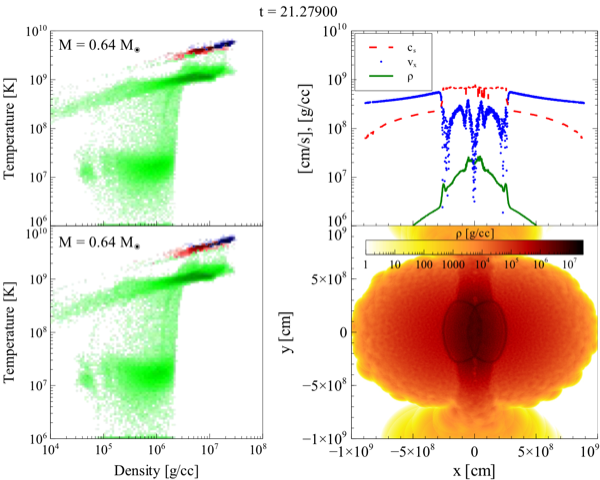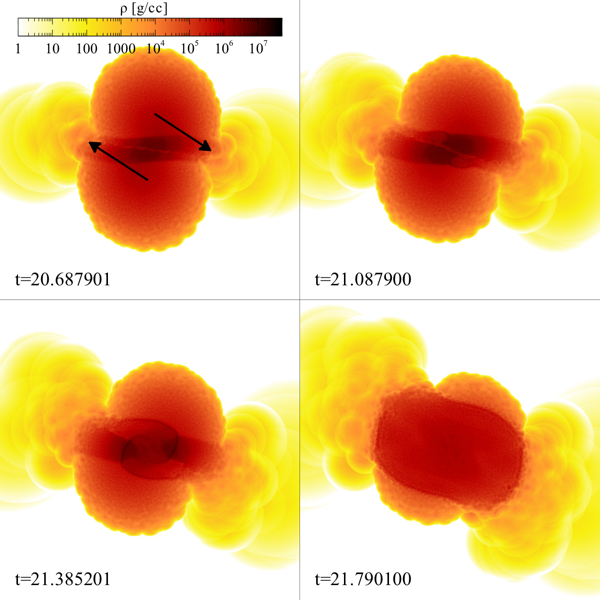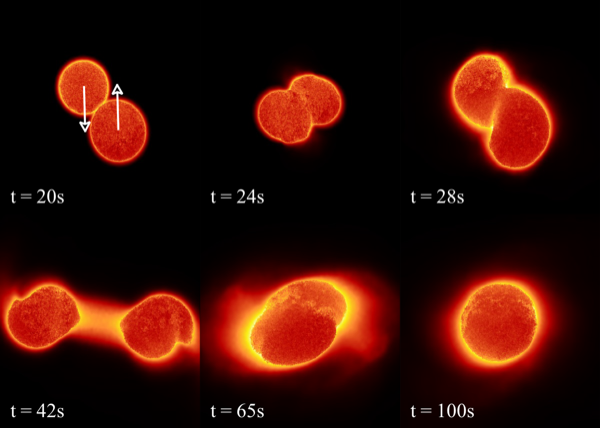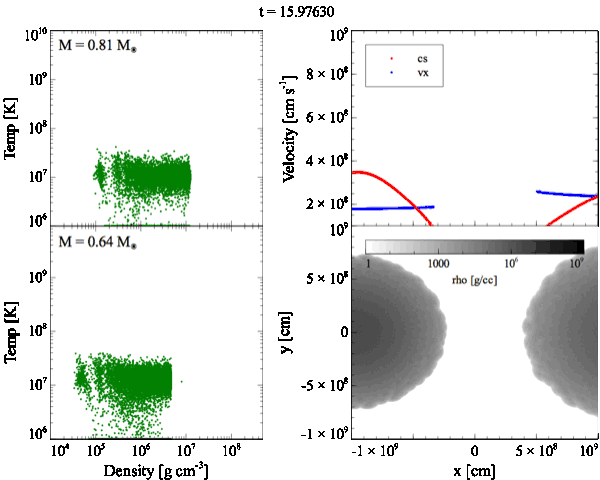0.6x2comb.mp4, 0.6_0.8comb.mp4, 0.6_1.0comb.mp4On Type Ia supernovae from the collisions of two white dwarfs (collisions I, 2009)
In this letter we explore collisions between two white dwarfs as a pathway for making Type Ia supernovae (SNIa). White dwarf number densities in globular clusters allow 10-100, redshift z $\lesssim$ 1 collisions per year, and observations by Chomiuk et al. of globular clusters in the nearby S0 galaxy NGC 7457 have detected what is likely to be a SNIa remnant. We carry out simulations of the collision between two 0.6 M$_{\odot}$ white dwarfs at various impact parameters and mass resolutions. For impact parameters less than half the radius of the white dwarf, we find such collisions produce $\simeq$ 0.4 M$_{\odot}$ of $^{56}$Ni, making such events potential candidates for underluminous SNIa or a new class of transients between Novae and SNIa.